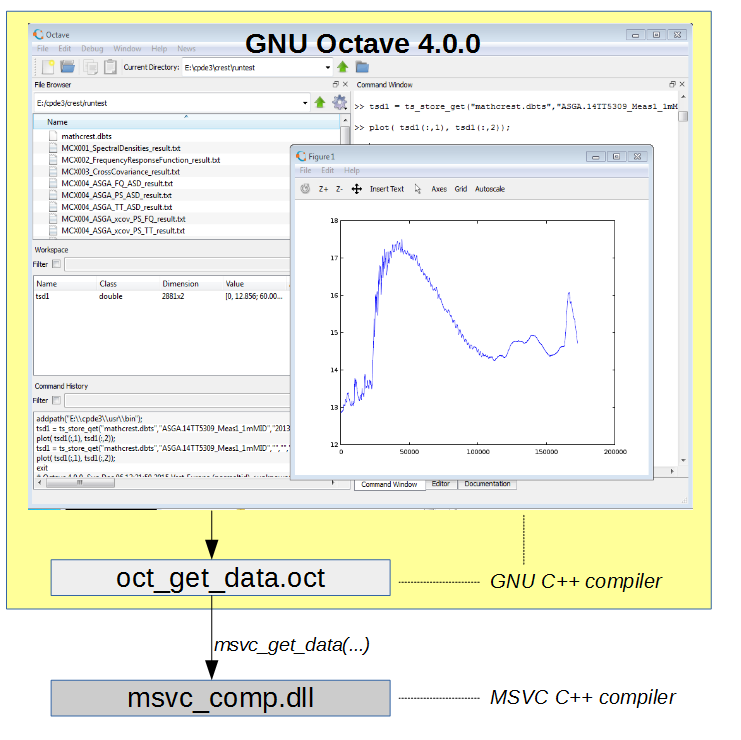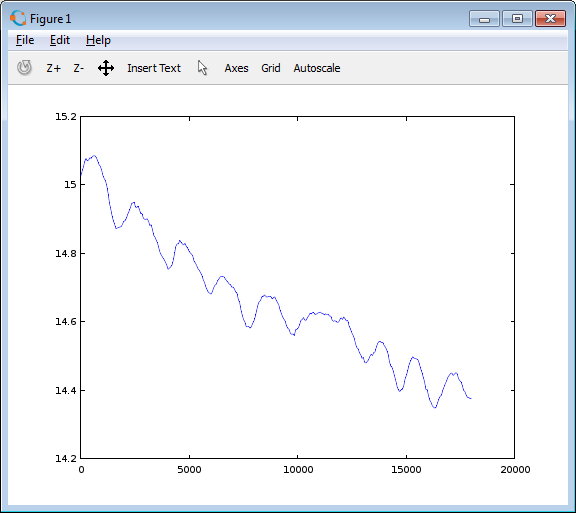# Octave plug-in calling MSVC

This post is about creating plugins to the Windows version of GNU Octave 4.0.0 (Octave) using existing components created with Microsoft Visual Studio 2013 (MSVC). It takes too long to explain why this is sometimes useful, just assume that it is. A typical scenario could be that you have some kind of database accessible from MSVC code and you want to expose the data in Octave.

The figure below illustrates a possible setup. To implement a plugin in Octave, you write a piece of C++ code and compile/link it into a special kind of shared library referred to as an 'oct-file'. Such code can call other components, for example a DLL (Dynamic Link Library) created with MSVC. This way, the oct-file functions as the glue between the Octave application and some other software component.T
his sounds simple enough, but in practice there are a couple of things to handle to make it work:

First, Octave and its oct-files are compiled using the MinGW GNU g++ C++ compiler (It is not practical to recompile Octave using MSVC) and GNU g++ code is not binary compatible with MSVC code. Therefore, we cannot statically link the MSVC dll with the oct-file and we cannot pass C++ objects in the calls between them, because name mangling schemes and calling conventions are incompatible between the compilers.

Second, Octave and its oct-files are compiled as 32bit. Even if we find a way around the first problem, it will not work if the MSVC component is compiled as 64bit, it has to be 32bit as Octave.

Now the whole thing sounds a lot more complicated, but the problem description also provides the clues to the solution

• Use a C interface in the calls from the oct-file (GNU g++) to the MSVC code

• Load the MSVC dll dynamically instead of linking statically

• Compile the MSVC code as 32 bit

### MSVC C++ code

The first bullet above means we must provide global functions declared as extern ”C” in the MSVC C++ code (linked as DLL, and exported), here is a sample header declaration:

#ifndef MSVC_COMP_H
#define MSVC_COMP_H

#ifdef MSVC_COMP_IMPLEMENTATION

#define MSVC_COMP_PUBLIC __declspec(dllexport)

#else

#define MSVC_COMP_PUBLIC __declspec(dllimport)

#endif

extern "C" {

// msvc_get_data returns a pointer to internal data, must not be deleted outside

MSVC_COMP_PUBLIC double* msvc_get_data(const char* file_path, const char* data_id, long* nsamp);

}

#endif // MSVC_COMP_H

Note that the function takes and returns parameters as if it was an old style C-function, no C++ objects or pointers are allowed, du to the compiler differences. The extern ”C” statement removes any name mangling in the compiled name, it makes it possible to look up from other code (see below).

Notice also that in this case, the function returns a pointer to some numerical data. Such data may be dynamically allocated by the MSVC compiler, and cannot be deleted in the GNU code (in this case the pointer ponts to a global variable internally in the MSVC code and it will be cleaned up in the next call).

The above is just the header file declaration, the implementation of the msvc_get_data function can use all C++ constructs, objects, pointers etc.  The msvc_get_data function is thus just an adapter.

### OCT-file code

The second bullet in our list, and how the OCT-file interfaces the MSVC code is best illustrated using an example (note that most error checking has been omitted for clarity).

File oct_get_data.cpp:

#include <octave/oct.h>

#include <fstream>

#include <windows.h>

#include <dMatrix.h>

// function pointer to the MSVC fuunction

typedef double* (*msvc_gdfunc)(const char* file_path, const char* data_id, long* nsamp);

// Octave function declaration

DEFUN_DLD (oct_get_data, args, nargout, "oct_get_data String")

{

int nargin = args.length ();

if(nargin < 2) {

octave_stdout << "oct_get_data called with "

<< nargin << " input and "

<< nargout << " output arguments.\n";

octave_stdout << "Usage:  oct_get_data(file_path,data_id); \n";

}

// get the arguments from the Octave call

int iarg=0;

std::string file_path   = args(iarg++).char_matrix_value().row_as_string(0);

std::string data_id     = args(iarg++).char_matrix_value().row_as_string(0);

// load the MSVC DLL dynamically using Windows API

HMODULE hdll = LoadLibrary("msvc_comp.dll");

if(hdll != NULL) {

// get the function pointer

msvc_gdfunc msvc_get_data = (msvc_gdfunc)GetProcAddress(hdll,"msvc_get_data");

if(msvc_get_data) {

// call the function in the MSVC dll

long nsamp = 0;

if(double* values = msvc_get_data(file_path.c_str(),data_id.c_str(),&nsamp)) {

// assign output data, it returns data column major order, 2 columns

Matrix result(nsamp,2);

for(int j=0;j<2;j++) {

for(int i=0; i<nsamp; i++) {

result(i,j) = *values++;

}

}

return octave_value(result);

}

}

}

// empty return value

return octave_value_list();

}

The code above declares a function pointer type for our MSVC function. It then uses the Windows API to load the DLL dynamically + look up the pointer to the function. If found, it calls the MSVC function and constructs a suitable matrix object to return to the Octave application.

Obviously much more rigorous error checking is in order.

### Compiling the OCT-file

As previously mentioned, the oct-file must be compiled using the GNU C++ compiler to be compatible with Octave. For this purpose we use the mkoctfile utility. It can be done within Octave, or via a Wiindows batch script as shown below.

File cppoct.bat:

@echo off

REM Script to compile C++ into Octave oct-files

REM

REM configure Octave oct compiler

set OCT_VER=4.0.0

set OCT_HOME=C:\Octave\Octave-%OCT_VER%

set OCT_BIN=%OCT_HOME%\bin

set OCT_INC=%OCT_HOME%\include\octave-%OCT_VER%

set OCT_LIB=%OCT_HOME%\lib\octave\%OCT_VER%

REM

REM set Octave bin dir first in path so g++ can be found

set PATH=%OCT_BIN%;%PATH%

REM

REM turn on echo so we can see what is going on as we compile

@echo on

%OCT_BIN%\mkoctfile.exe -I%OCT_INC% -I%OCT_INC%\octave  -L%OCT_LIB%  %1

@echo off

REM tidy up intermediate files

del *.o

Running this script for the oct_get_data.cpp file generates the oct_get_data.oct file.

### Using the plugin in Octave

Once the oct-file and the msvc dll exist, it is recommended to store them in a common folder in the file system. In Octave, you then need to specify that folder using 'addpath'.

addpath("C:\\somefolder");
data = oct_get_data("myfile.dat","whatever");
plot( data(:,1), data(:,2));

Assuming the data returned was a matrix of X,Y data, the result could look something like belowIf you find this useful, please add a comment below :-)